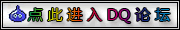## 基础计算公式

DQ9的基础伤害计算和之前的DQ系列一样，

[伤害计算值]=[伤害基础值]=攻击力/2-防御力/4

[伤害波动]=[伤害计算值]/16+1

[实际伤害值]=(攻击力/2-防御力/4)±[伤害波动]
(实际伤害始终是这个公式，下面不再重复列出)

## 辅助咒语影响

[伤害计算值]=[伤害基础值]=攻击力×攻击倍率/2-防御力×防御倍率/4

ルカナン与スクルト只有范围增加，效果并没有增加

## 武器对敌特效影响

[伤害计算值]=[伤害基础值]×特效倍率

## 元素属性影响

[伤害计算值]=[伤害基础值]×特效倍率×属性倍率

## 技能影响

[伤害计算值]=[伤害基础值]×特效倍率×属性倍率×技能倍率

## 其他影响

[伤害计算值]=[伤害基础值]×特效倍率×属性倍率×技能倍率×其他倍率

5:[伤害计算值]=([伤害基础值]×1.5＋(等级/10+1)×1)×特效倍率×技能倍率×其他倍率
20:[伤害计算值]=([伤害基础值]×2.5＋(等级/10+1)×2)×特效倍率×技能倍率×其他倍率
50:[伤害计算值]=([伤害基础值]×4.0＋(等级/10+1)×3)×特效倍率×技能倍率×其他倍率
100:[伤害计算值]=([伤害基础值]×6.0＋(等级/10+1)×4)×特效倍率×技能倍率×其他倍率

## 综合

[伤害计算值]=((攻击力×攻击倍率/2-防御力×防御倍率/4)×蓄力倍率)+(等级/10+1)×蓄力次数)×特效倍率×属性倍率×技能倍率×其他倍率
[伤害波动]=[伤害计算值]/16+1
[实际伤害值]=[伤害计算值]±[伤害波动]